Next: Exercises Up: Newton's Laws of Motion Previous: Newton's Third Law of

Non-Isolated Systems

Up to now, we have only considered isolated dynamical systems, in which all of the forces acting on the system originate within the system itself. Let us now generalize our approach to deal with non-isolated dynamical systems, in which some of the forces originate outside the system. Consider a system of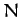mutually interacting point objects. Let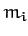and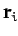be the mass and position vector of theth object, respectively. Suppose that theth object is subject to two forces. First, an internal force which originates from the other objects in the system, and second an external force which originates outside the system. In other words, let the force acting on theth object take the form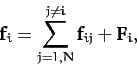(38)

where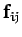is the internal force exerted by object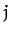on object, and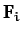the net external force acting on object.

The equation of motion of theth object is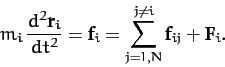(39)

Summing over all objects, we obtain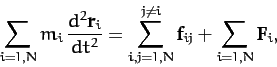(40)

which reduces to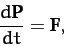(41)

where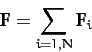(42)

is the net external force acting on the system. Here, the sum over the internal forces has cancelled out in pairs due to Newton's third law of motion. We conclude that the total system momentum evolves in time according to the simple equation (41) when there is a net external force acting on the system, but is completely unaffected by the internal forces. The fact that Equation (41) is similar in form to Equation (13) suggests that the center of mass of a system of many point objects has analogous dynamics to a point object.

Taking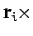Equation (39), and summing over all objects, we obtain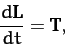(43)

where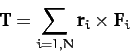(44)

is the net external torque acting on the system. Here, the sum over the internal torques has cancelled out in pairs, assuming that the internal forces are central in nature. We conclude that the total system angular momentum evolves in time according to the simple equation (43) when there is a net external torque acting on the system, but is completely unaffected by the internal torques.Next: Exercises Up: Newton's Laws of Motion Previous: Newton's Third Law of
Richard Fitzpatrick 2011-03-31